# Multiplication

1
One factor is 0
2
One factor is 1
3
One factor is 2
4
One factor is 3
5
One factor is 1-3
6
One factor is 4
7
One factor is 5
8
One factor is 6
9
One factor is 4-6
10
One factor is 7
11
One factor is 8
12
One factor is 9
13
One factor is 10
14
One factor is 7-10
15
One factor is 2-9
16
One factor is 1-10
17
One factor is 11
18
One factor is 12
19
One factor is 13
20
One factor is 11-13
21
One factor is 14
22
One factor is 15
23
One factor is 16
24
One factor is 14-16
25
One factor is 17
26
One factor is 18
27
One factor is 19
Difficult
28
One factor is 20
Difficult
29
One factor is 17-20
30
One factor is 11-20
31
One factor is 21-25
32
One factor is 26-30
33
One factor is 31-35
Difficult
34
One factor is 36-40
35
One factor is 41-45
36
One factor is 46-50
37
One factor is 51-55
38
One factor is 56-60
39
One factor is 61-65
40
One factor is 66-70
41
One factor is 71-75
42
One factor is 76-80
43
One factor is 81-85
Difficult
44
One factor is 86-90
45
One factor is 91-100
Difficult

## Recent activities in this game

Someone got all questions correct at level 5. One factor is 1-3 .
4 hours ago after 26 seconds of playing.
Someone got all questions correct at level 4. One factor is 3 .
4 hours ago after 1 minute of playing.
Someone got all questions correct at level 3. One factor is 2 .
4 hours ago after 25 seconds of playing.
Someone got all questions correct at level 2. One factor is 1 .
4 hours ago after 26 seconds of playing.
Someone got all questions correct at level 1. One factor is 0 .
4 hours ago after 47 seconds of playing.
Someone had a problem at level 3. One factor is 2 .
10 hours ago after 4 seconds of playing.
Someone got all questions correct at level 11. One factor is 8 .
12 hours ago after 1 minute of playing.
Someone had a problem at level 11. One factor is 8 .
12 hours ago after 2 minutes of playing.
Someone got all questions correct at level 10. One factor is 7 .
13 hours ago after 2 minutes of playing.
Someone got all questions correct at level 8. One factor is 6 .
13 hours ago after 16 minutes of playing.

Get better at multiplication. Do you know how to multiply and find the products or multiples of these factors?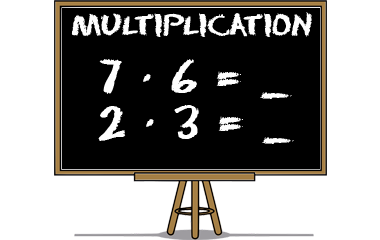## Game instructions for multiplication

Choose a number from the buttons above. This will give you a game with up to ten questions where you have to multiply one number (factor 1) with another number (factor 2) and find the product. If you can solve all the tasks, you will get a knowledge point and the numbers button will be marked green.

### Multiplication in the language of mathematics

Multiplication is one of the four basic rules of arithmetic and some say that they are counting times. The correct term is that they are multiplying. You often use a multiplication table, or times table, while learning to multiply.

One factor times another factor results in a product:
 3 · 2 = 6 factor · factor = product

### Times table chart

Below is the multiplication chart, or times table chart, that helps the student to multiply by selecting column and row and then see the product of the two factors. In the chart below we have put together all multiplication tables, from 0 times table up to 10 times table.
·012345678910
000000000000
1012345678910
202468101214161820
3036912151821242730
40481216202428323640
505101520253035404550
606121824303642485460
707142128354249566370
808162432404856647280
909182736455463728190
100102030405060708090100

### Calculation of knowledge points

Each completed game level gives 1 knowledge point in Multiplication. The maximum number of points (45 knowledge points) is achieved when you pass all 45 levels. You'll get a bronze medal when you complete a level 2 times and a silver medal after 5 completed rounds. A gold medal will be received after 10 completed rounds. In Multiplication, the maximum number of collectable gold medals is 45. You only earn knowledge points for levels that has not been cleared before. A cleared level has a green background above. Even when you have completed a level, you can continue practicing at that level, but it gives you no more knowledge points.

### Similar games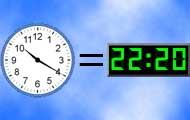Math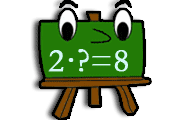Math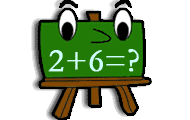MathMath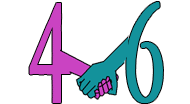Math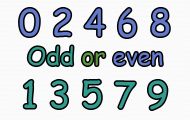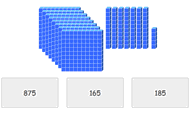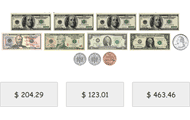Math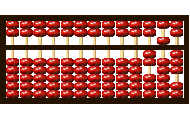Math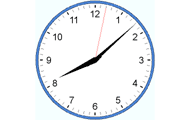Subject
Math
Target group
Practices
Numbers Multiplication Mental calculation
Game creator
Updated
2019-09-30
Published online
2011-11-11
Unique id
9
Saved by
5-10 users
Operating system
Browser
Price
Free of charge

4.1
Total 746
5
518
4
41
3
52
2
37
1
98

### Report

If you find a spelling mistake or something else that should be corrected, feel free to contact the game creator or us at Helpful Games.

Write a comment below to contact the game creator or

(Not shown - only for responses)
There are a total of 11 comments on this knowledge game.

A
25 September 2023 20:36
I think that this game would be good to use if your having a problem with some sorts multiplications.And it would be good to use if you love math as much as me.I recommend this.You should try it out.
E
20 September 2022 19:54
Hi everyone! I like this game and I think level 34 is kinda hard for me.so i think i should practice more! I give this game 3/5!!!
3 December 2021 16:39
There are 45 levels. If you press the Give up, then it would say '' No knowledge points this time!
B
19 April 2021 20:57
Pretty good it could use some games though otherwise good
F
17 April 2021 12:00
very good sooo much fun. i love math
L
4 May 2020 09:28
😎 cool love multiplication llll
S
7 March 2019 08:44
It's very easy to learn multitlication! :)
3 March 2017 15:33
Best free game to practice multiplication
R
24 February 2017 19:28
Very educating i think.
J
16 February 2017 23:29
Math is fun now. Love this!
7 September 2016 16:52
this is good game yes i,like multiply
Most played
1.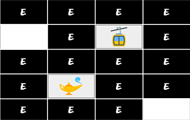Brain training
2.Brain training
3.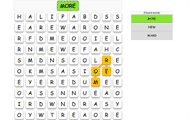English
4.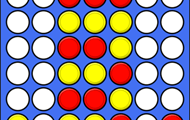Brain training
5.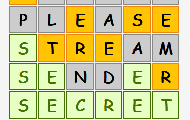English